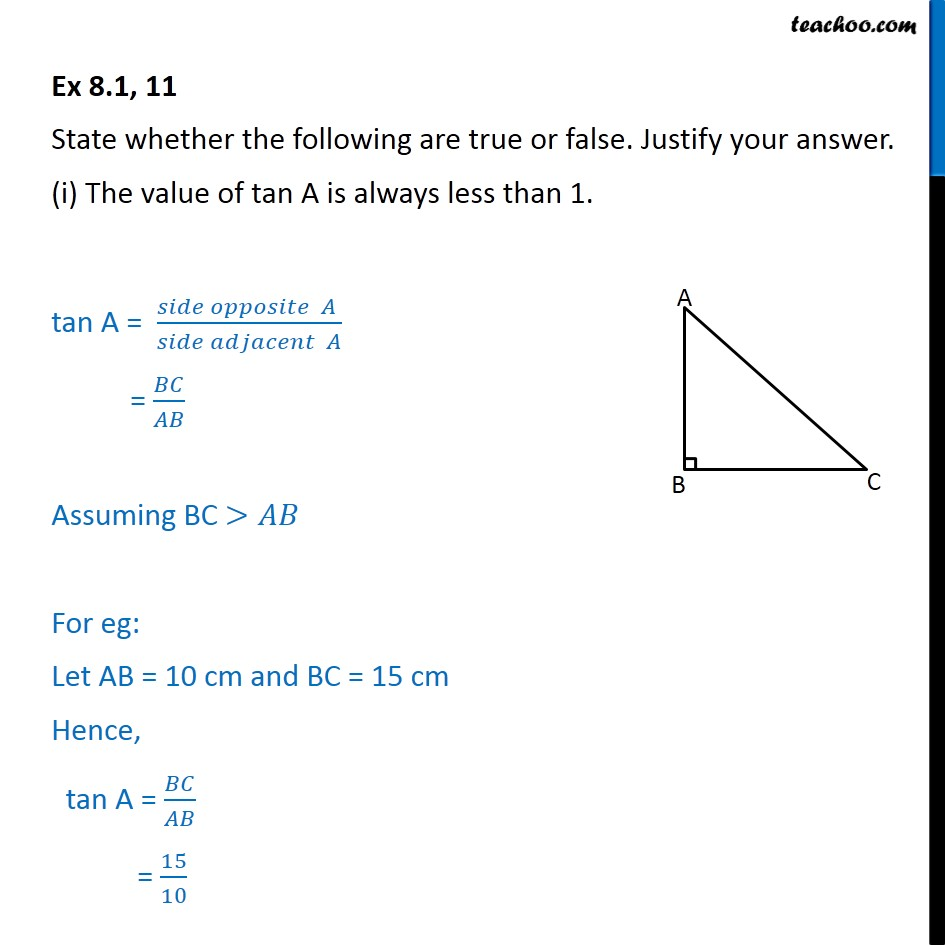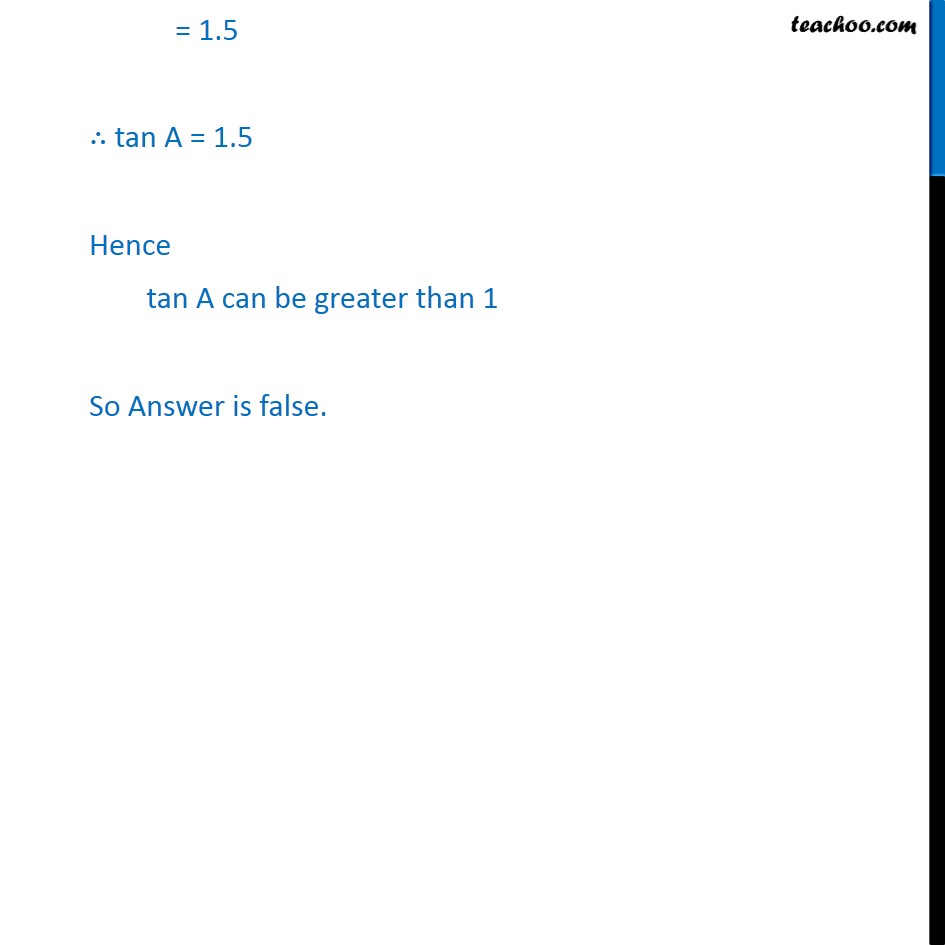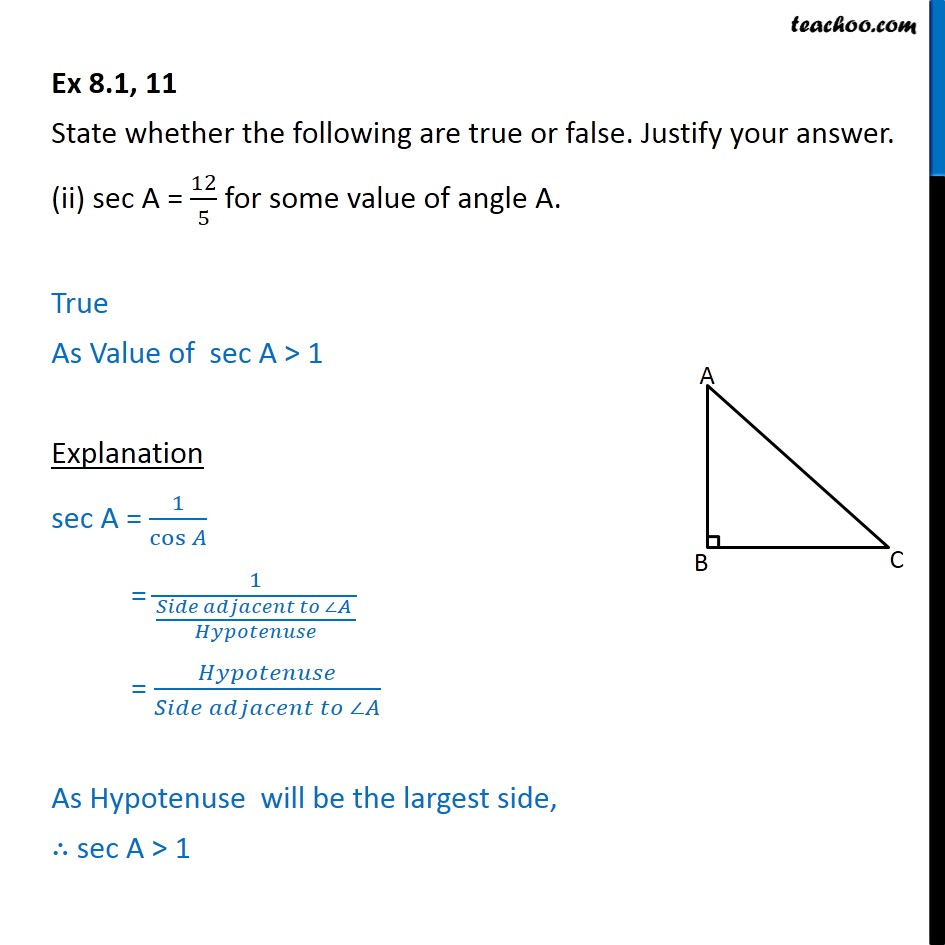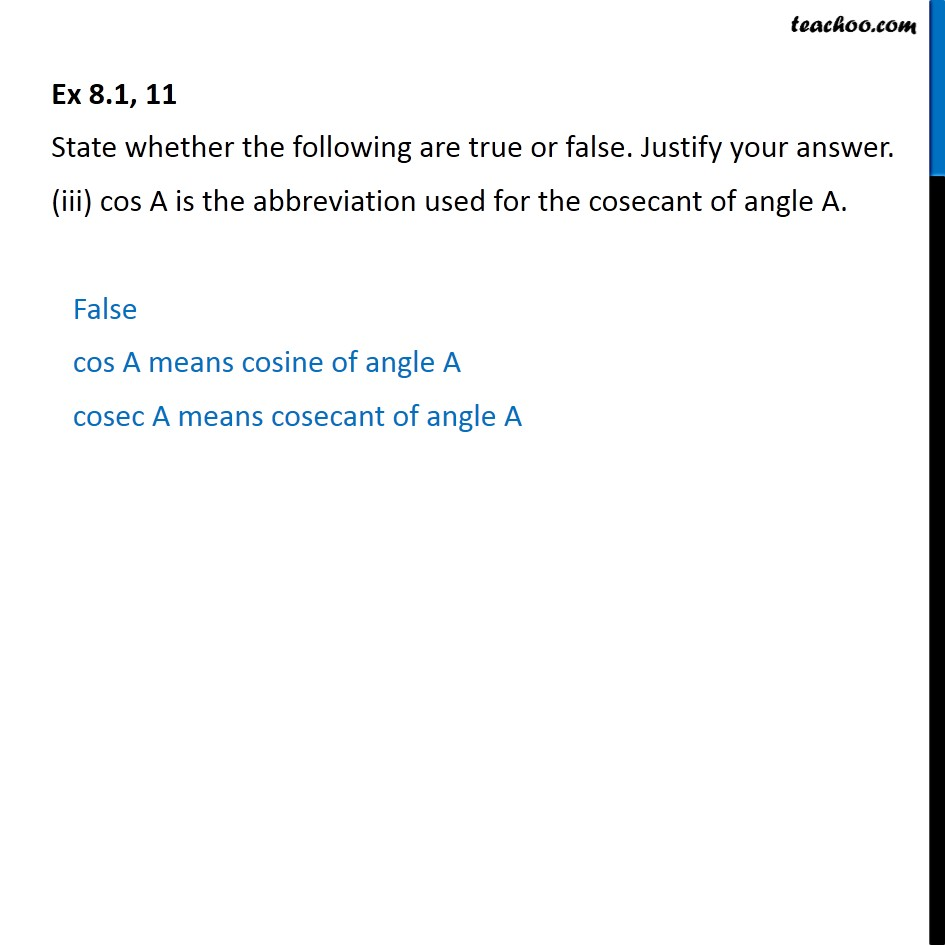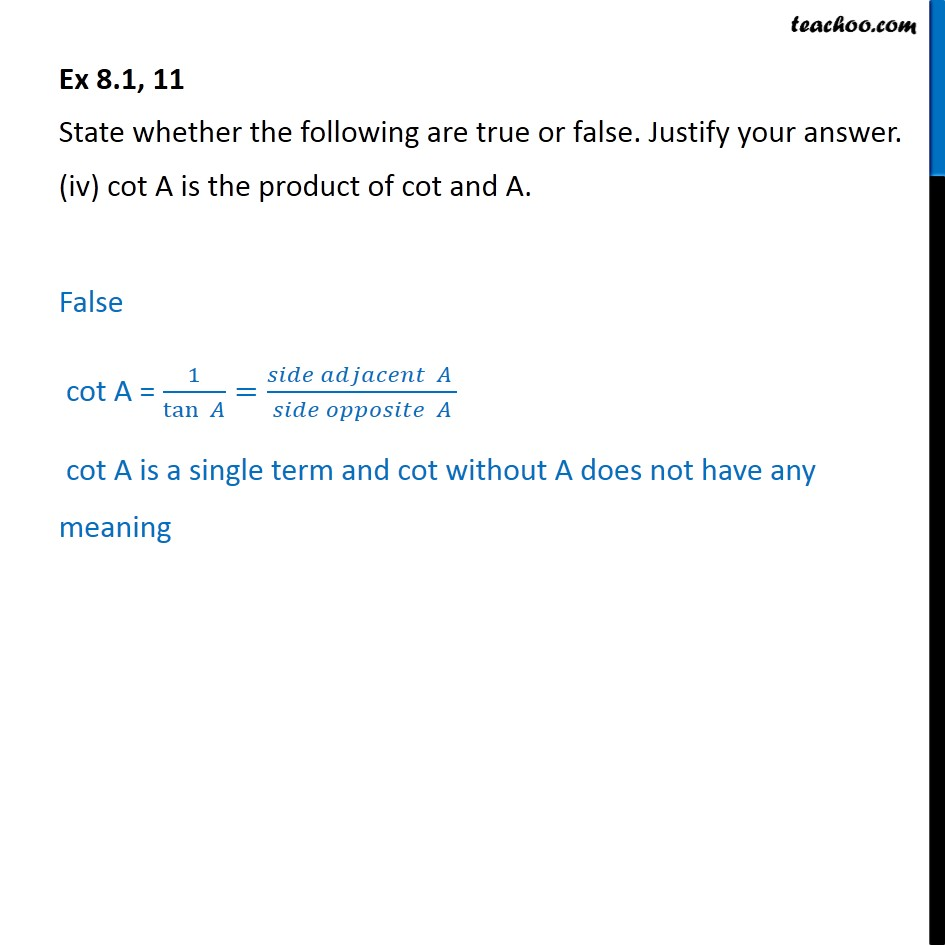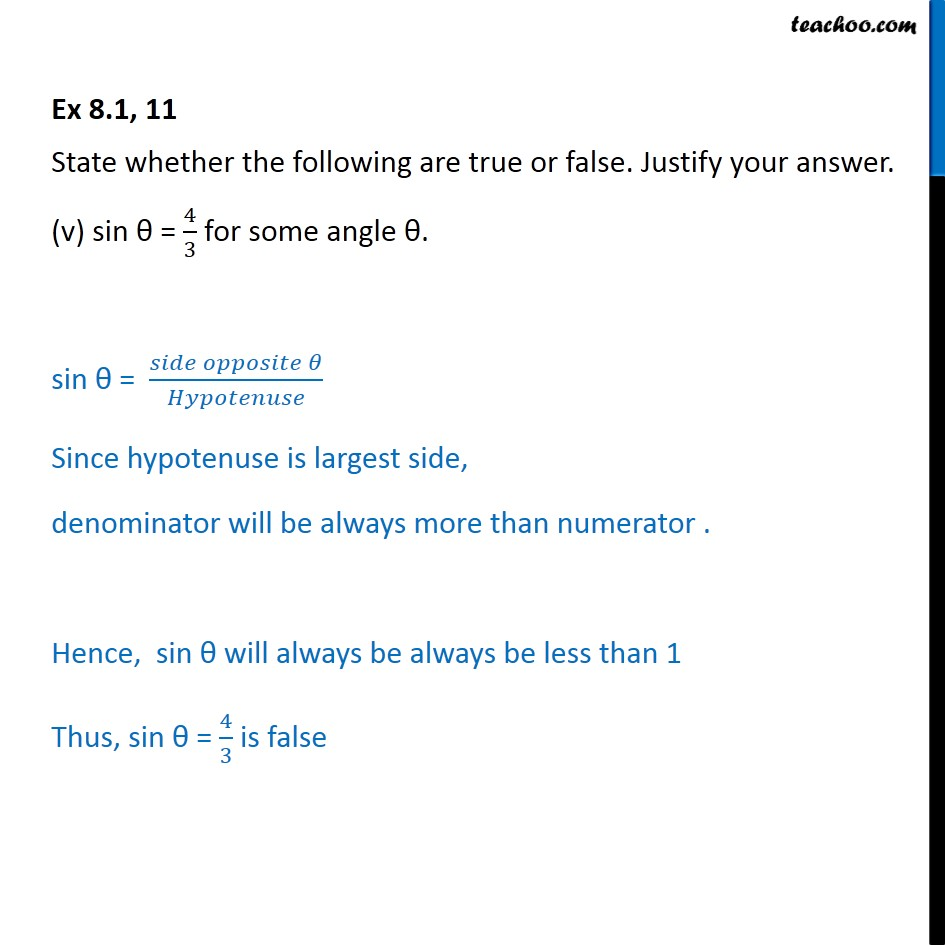1. Chapter 8 Class 10 Introduction to Trignometry (Term 1)
2. Serial order wise
3. Ex 8.1

Transcript

Ex 8.1, 11 State whether the following are true or false. Justify your answer. (i) The value of tan A is always less than 1. State whether the following are true or false. Justify your answer. (ii) sec A = 12/5 for some value of angle A. Ex 8.1, 11 State whether the following are true or false. Justify your answer. (iii) cos A is the abbreviation used for the cosecant of angle A. False cos A means cosine of angle A cosec A means cosecant of angle A